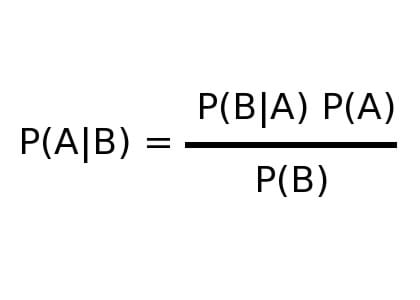﻿

# 蒙提霍爾問題模擬運算 Monty Hall Problem Simulation## 用貝氏定理來解決條件機率問題1. P(A|B)：在B事件已經發生的情況下，A事件發生的機率。
2. P(B|A)：在A事件已經發生的情況下，B事件發生的機率。
3. P(A)：沒有任何前提下，A發生的機率。
4. P(B)：沒有任何前提下，B發生的機率。

P(A|B) = (1/2 * 1/3) / 1/2 = 1/3，也就是說，如果你堅持不換門的話，抽到汽車的機率只有1/3，如果換門的話，可以提升到2/3。這是貝氏定理跟我們講的事情，我相信大部的人的還是堅持無論如何機率都是1/2。沒錯，這是我們大腦依據最短路徑跟我們講的結果，所以下面我們就開始寫一隻程式來跑模擬吧！

## Monty Hall Problem Simulation 蒙提霍爾問題模擬運算

Please input numbers to simulate and click "Experimentation" button. 請在下方輸入要模擬實驗（丟骰子）的次數，然後按下實驗按鈕後，就可以看出亂數模擬出來的結果。

Simulate times.

## 實驗結果

• 無論你覺得自己多麼的聰明，眼見不一定為憑，你當下用腦思考的深思熟慮也不一定是最正確的決定。
• 世事無絕對！你就算你經過千百次計算佐以數據證明之事，未必適用於他人。在時空中的某一點你們相遇，未必代表在這點之前，你們的遭遇或經歷是相同的，因此就算你們一起站在同一個時空時間點上，大家的觀點與立場也未必相同。
• 條件機率告訴我們，不要去輕易地批判他人的決定。
• 條件機率告訴我們，輕易地批判他人決定的人，該人的世界觀一定很狹隘。

## Monty Hall Problem Simulation with JavaScript Source Code

You can also view HTML/JavaScript source code of this webpage.

``````<html>
<meta http-equiv="content-type" content="text/html; charset=utf-8">
<script src="http://code.jquery.com/jquery-2.1.4.min.js"></script>
<title>Monty Hall Problem Simulation with JavaScript Source Code</title>
<body>
<div id="probabilityForSwitch"></div>
<div id="probabilityForNonSwitch"></div>

<script>
//亂數種子函式(seedrandom.min.js)
!function(r,n,t,e,o,i,u,a,f){function c(r){var n,t=r.length,o=this,i=0,u=o.i=o.j=0,a=o.S=[];for(t||(r=[t++]);e>i;)a[i]=i++;for(i=0;e>i;i++)a[i]=a[u=m&u+r[i%t]+(n=a[i])],a[u]=n;(o.g=function(r){for(var n,t=0,i=o.i,u=o.j,a=o.S;r--;)n=a[i=m&i+1],t=t*e+a[m&(a[i]=a[u=m&u+n])+(a[u]=n)];return o.i=i,o.j=u,t})(e)}function p(r,n){var t,e=[],o=typeof r;if(n&&"object"==o)for(t in r)try{e.push(p(r[t],n-1))}catch(i){}return e.length?e:"string"==o?r:r+"\x00"}function l(r,n){for(var t,e=r+"",o=0;o<e.length;)n[m&o]=m&(t^=19*n[m&o])+e.charCodeAt(o++);return g(n)}function s(t){try{return h?g(h.randomBytes(e)):(r.crypto.getRandomValues(t=new Uint8Array(e)),g(t))}catch(o){return[+new Date,r,(t=r.navigator)&&t.plugins,r.screen,g(n)]}}function g(r){return String.fromCharCode.apply(0,r)}var h,y=t.pow(e,o),d=t.pow(2,i),v=2*d,m=e-1,j=t["seed"+f]=function(r,i,u){var a=[];i=1==i?{entropy:!0}:i||{};var h=l(p(i.entropy?[r,g(n)]:null==r?s():r,3),a),m=new c(a);return l(g(m.S),n),(i.pass||u||function(r,n,e){return e?(t[f]=r,n):r})(function(){for(var r=m.g(o),n=y,t=0;d>r;)r=(r+t)*e,n*=e,t=m.g(1);for(;r>=v;)r/=2,n/=2,t>>>=1;return(r+t)/n},h,"global"in i?i.global:this==t)};if(l(t[f](),n),u&&u.exports){u.exports=j;try{h=require("crypto")}catch(w){}}else a&&a.amd&&a(function(){return j})}(this,[],Math,256,6,52,"object"==typeof module&&module,"function"==typeof define&&define,"random");
//設定亂數種子
Math.seedrandom();
//總共實驗次數
var iLoop = 1000000;
//在交換演算法中猜對的次數
var iSwitchCount = 0;
//在非交換演算法中猜對的次數
var iNonSwitchCount = 0;
//開始模擬
for(i=0; i<iLoop; i++) {
//亂數指定[0,1,2]哪個門後是車子
var iAnswer = Math.floor(Math.random() * 3);
//亂數產生使用者指出[0,1,2]哪道門
var iGuest = Math.floor(Math.random() * 3);
//主持人知道答案，因此故意去開[0,1,2]一定是羊的門
var iHost;
while(true) {
iHost = Math.floor(Math.random() * 3);
if((iHost != iGuest) && (iHost != iAnswer)) { break; }
}
//計算結果（非交換）
if(iGuest == iAnswer) { iNonSwitchCount++; }
//改採用交換法則
switch(iHost) {
case 0:
if(iGuest == 1) { iGuest = 2; }
else { iGuest = 1; }
break;
case 1:
if(iGuest == 0) { iGuest = 2; }
else { iGuest = 0; }
break;
case 2:
if(iGuest == 0) { iGuest = 1; }
else { iGuest = 0; }
break;
}
//計算結果（交換）
if(iGuest == iAnswer) { iSwitchCount++; }
}
\$("#probabilityForSwitch").text((iSwitchCount / iLoop) * 100);
\$("#probabilityForNonSwitch").text((iNonSwitchCount / iLoop) * 100);
</script>
</body>
</html>
``````